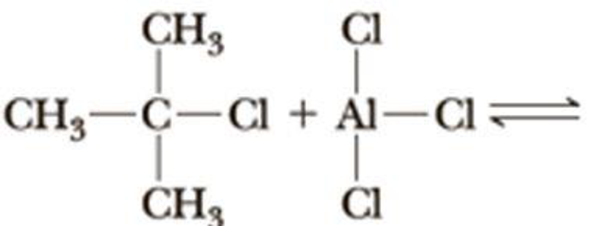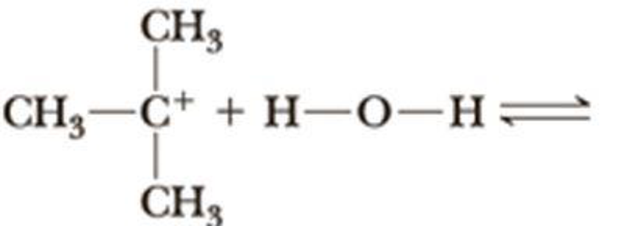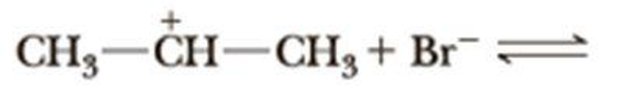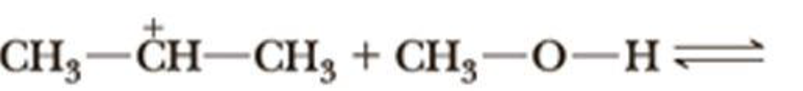# Complete the equation for the reaction between each Lewis acid-base pair. In each equation, label which starting material is the Lewis acid and which is the Lewis base; use curved arrows to show the flow of electrons in each reaction. In doing this problem, it is essential that you show valence electrons for all atoms participating in each reaction. (a) (b) (c) (d)### Organic Chemistry

8th Edition
William H. Brown + 3 others
Publisher: Cengage Learning
ISBN: 9781305580350

#### Solutions

Chapter
Section### Organic Chemistry

8th Edition
William H. Brown + 3 others
Publisher: Cengage Learning
ISBN: 9781305580350
Chapter 4, Problem 4.33P
Textbook Problem
147 views

## Complete the equation for the reaction between each Lewis acid-base pair. In each equation, label which starting material is the Lewis acid and which is the Lewis base; use curved arrows to show the flow of electrons in each reaction. In doing this problem, it is essential that you show valence electrons for all atoms participating in each reaction.(a)(b)(c)(d)(a)

Interpretation Introduction

Interpretation:

Using curved arrows to indicate flow of electrons, a reaction equation between 2-chloro-2-methylpropane and aluminum trichloride has to be given and label the Lewis acid and the Lewis base in the reactants.

Concept Introduction:

Lewis acid:  These are substance that donates proton and accepts a pair of nonbonding electrons.

Lewis base: These are substances that accept proton and donate a pair of nonbonding electrons .

Conjugate acid:  It is formed when a base accepts a proton from an acid.

Conjugate base:  Transformation of proton from an acid to a base leads to formation of new species called conjugate base.

Lewis structure: This is only way to show the sharing of electrons between atom in polar covalent bonds or covalent bond.  This is often called Lewis structure.  This structure denotes valence electrons of an atom by dots and also it shows the way of valence electron distributed within a molecule.

### Explanation of Solution

2-chloro-2-methylpropane reacts with aluminum trichloride in which lone pair of electrons of chlorine atom in 2-chloro-2-methylpropane attack aluminum to form a complex ion...

(b)

Interpretation Introduction

Interpretation:

Using curved arrows to indicate flow of electrons, a reaction equation between a tertiary carbocation and water has to be given and label the Lewis acid and the Lewis base in the reactants.

Concept Introduction:

Lewis acid:  These are substance that donates proton and accepts a pair of nonbonding electrons.

Lewis base: These are substances that accept proton and donate a pair of nonbonding electrons .

Conjugate acid:  It is formed when a base accepts a proton from an acid.

Conjugate base:  Transformation of proton from an acid to a base leads to formation of new species called conjugate base.

Lewis structure: This is only way to show the sharing of electrons between atom in polar covalent bonds or covalent bond.  This is often called Lewis structure.  This structure denotes valence electrons of an atom by dots and also it shows the way of valence electron distributed within a molecule.

(c)

Interpretation Introduction

Interpretation:

Using curved arrows to indicate flow of electrons, a reaction equation between a secondary carbocation and bromide ion has to be given and label the Lewis acid and the Lewis base in the reactants.

Concept Introduction:

Lewis acid:  These are substance that donates proton and accepts a pair of nonbonding electrons.

Lewis base: These are substances that accept proton and donate a pair of nonbonding electrons.

Conjugate acid:  It is formed when a base accepts a proton from an acid.

Conjugate base:  Transformation of proton from an acid to a base leads to formation of new species called conjugate base.

Lewis structure: This is only way to show the sharing of electrons between atom in polar covalent bonds or covalent bond.  This is often called Lewis structure.  This structure denotes valence electrons of an atom by dots and also it shows the way of valence electron distributed within a molecule.

(d)

Interpretation Introduction

Interpretation:

Using curved arrows to indicate flow of electrons, a reaction equation between a secondary carbocation and methanol has to be given and label the Lewis acid and the Lewis base in the reactants.

Concept Introduction:

Lewis acid:  These are substance that donates proton and accepts a pair of nonbonding electrons.

Lewis base: These are substances that accept proton and donate a pair of nonbonding electrons.

Conjugate acid:  It is formed when a base accepts a proton from an acid.

Conjugate base:  Transformation of proton from an acid to a base leads to formation of new species called conjugate base.

Lewis structure: This is only way to show the sharing of electrons between atom in polar covalent bonds or covalent bond.  This is often called Lewis structure.  This structure denotes valence electrons of an atom by dots and also it shows the way of valence electron distributed within a molecule.

### Still sussing out bartleby?

Check out a sample textbook solution.

See a sample solution

#### The Solution to Your Study Problems

Bartleby provides explanations to thousands of textbook problems written by our experts, many with advanced degrees!

Get Started

Find more solutions based on key concepts
What would happen if the squids environment gradually changedwould all squid die?

Oceanography: An Invitation To Marine Science, Loose-leaf Versin

The potential difference across the filament of a light-bulb is maintained at a constant value while equilibriu...

Physics for Scientists and Engineers, Technology Update (No access codes included)

Worldwide, of those who die each year from starvation and related illnesses are children, a three-fourths one-t...

Nutrition: Concepts and Controversies - Standalone book (MindTap Course List)

Understanding Nutrition (MindTap Course List)

How do a solute, a solvent, and a solution differ?

Biology: The Dynamic Science (MindTap Course List)

What evidence can you give that the corona has a very high temperature?

Horizons: Exploring the Universe (MindTap Course List)

The Willis Tower in Chicago is 1451 feet tall. How high is this in meters?

Introductory Chemistry: An Active Learning Approach